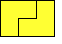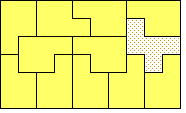# Tiling Rectangles with Pentominoes

Henri Picciotto

Some polyominoes can be used to tile rectangles. For example, here is the smallest rectangle that can be tiled by the bent tromino:1. The L, P and Y pentominoes can each tile a rectangle. What is the smallest possible such rectangle? Show each tiling on grid paper.
2. Tile a 3 by 5 rectangle with:
1. U and X
2. V and Z
3. Tile a 4 by 5 rectangle with:
1. T and Y
2. U and N
3. V and F
4. V and N
4. Tile a 5 by 5 square with:
1. X and Y
2. Y and Z
3. Y and F
4. L and X
5. Tile a 3 by 10 rectangle with:
1. U and Y
2. U and F
6. Find the smallest rectangle that can be tiled with
1. Y and N.
2. T and N.
3. T and W.

This figure shows a rectangle tiled with a single F pentomino, and many P's:1. What is the smallest rectangle you can tile with a single one of each of the pentominoes, and as many P's as you want?

This is an extension of page 27 in Polyomino Lessons.